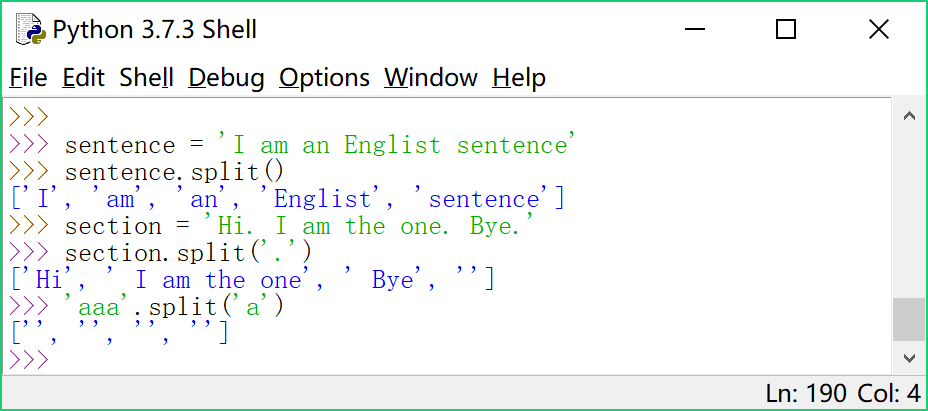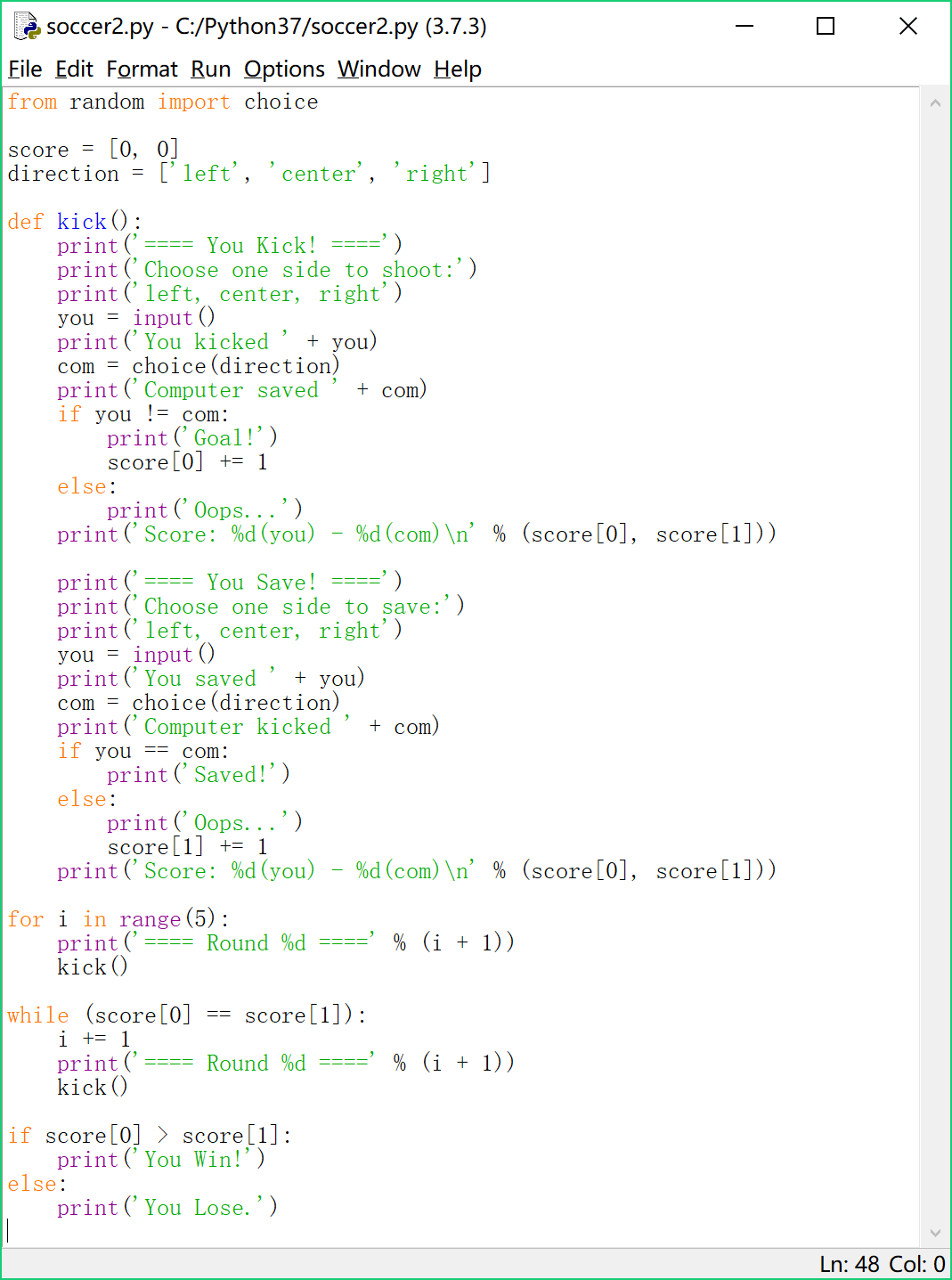## 【Python 第28课】字符串的分割

``sentence = 'I am an English sentence'``

``sentence.split()``

split() 会把字符串按照其中的空格进行分割，分割后的每一段都是一个新的字符串，最终返回这些字符串组成一个list。于是得到

``['I', 'am', 'an', 'English', 'sentence']``

``section = 'Hi. I am the one. Bye.'``

``section.split('.')``

``['Hi', ' I am the one', ' Bye', '']``

``'aaa'.split('a')``#==== 点球小游戏 ====#

``````from random import choice

score = [0, 0]
direction = ['left', 'center', 'right']

def kick():
print('==== You Kick! ====')
print('Choose one side to shoot:')
print('left, center, right')
you = input()
print('You kicked ' + you)
com = choice(direction)
print('Computer saved ' + com)
if you != com:
print('Goal!')
score += 1
else:
print('Oops...')
print('Score: %d(you) - %d(com)\n' % (score, score))

print('==== You Save! ====')
print('Choose one side to save:')
print('left, center, right')
you = input()
print('You saved ' + you)
com = choice(direction)
print('Computer kicked ' + com)
if you == com:
print('Saved!')
else:
print('Oops...')
score += 1
print('Score: %d(you) - %d(com)\n' % (score, score))

for i in range(5):
print('==== Round %d ====' % (i + 1))
kick()

while (score == score):
i += 1
print('==== Round %d ====' % (i + 1))
kick()

if score > score:
print('You Win!')
else:
print('You Lose.')``````